Next: Perihelion precession of planets Up: Orbits in central force Previous: Motion in general central

# Motion in nearly circular orbit

In principle, a circular orbit is a possible orbit for any attractive central force. However, not all such forces result in stable circular orbits. Let us now consider the stability of circular orbits in a general central force field. Equation (4.25) generalizes to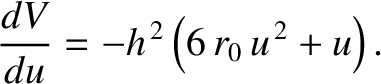(5.7)

where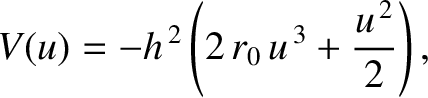is the radial force per unit mass. For a circular orbit,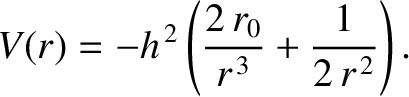, and the preceding equation reduces to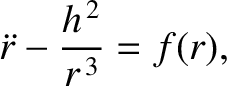(5.8)

where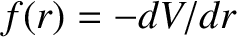is the radius of the orbit.

Let us now consider small departures from circularity. Suppose that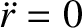(5.9)

Equation (5.7) can be written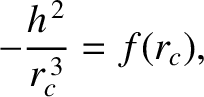(5.10)

Expanding the two terms involving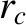as power series in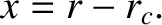, and keeping all terms up to first order, we obtain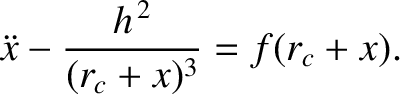(5.11)

where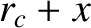denotes a derivative. Making use of Equation (5.8), the preceding equation reduces to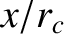(5.12)

If the term in square brackets is positive then we obtain a simple harmonic equation, which we already know has bounded solutions (see Section 2.8); in other words, the orbit is stable to small perturbations. On the other hand, if the term is square brackets is negative then we obtain an equation whose solutions grow exponentially in time (see Section 2.8); in other words, the orbit is unstable to small perturbations. Thus, the stability criterion for a circular orbit of radiusin a central force field characterized by a radial force (per unit mass) function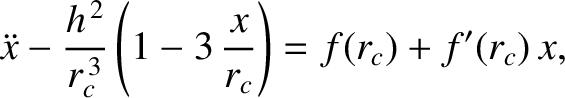is(5.13)

For example, consider an attractive power-law force function of the form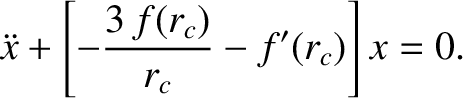(5.14)

where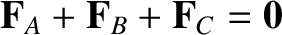. Substituting into the preceding stability criterion, we obtain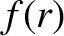(5.15)

or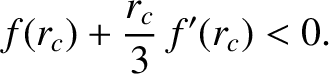(5.16)

We conclude that circular orbits in attractive central force fields that decay faster than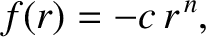are unstable. The case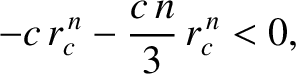is special, because the first-order terms in the expansion of Equation (5.10) cancel out exactly, and it is necessary to retain the second-order terms. Doing this, we can easily demonstrate that circular orbits are also unstable for inverse-cube () forces. (See Exercise 9.)

An apsis (plural, apsides) is a point on an orbit at which the radial distance,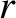, assumes either a maximum or a minimum value. Thus, the perihelion and aphelion points are the apsides of planetary orbits. The angle through which the radius vector rotates in going between two consecutive apsides is called the apsidal angle. Hence, the apsidal angle for elliptical orbits in an inverse-square force field is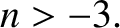.

For the case of stable, nearly circular orbits, we have seen thatoscillates sinusoidally about its mean value,. Indeed, it is clear from Equation (5.12) that the period of the oscillation is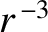(5.17)

The apsidal angle is the amount by which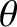increases in going between a maximum and a minimum of. The time taken to achieve this is clearly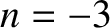. Now,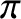, where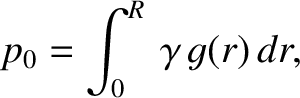is a constant of the motion, andis almost constant. Thus,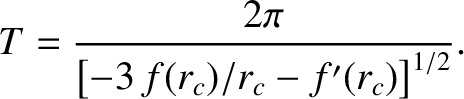is approximately constant. In fact,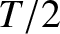(5.18)

where use has been made of Equation (5.8). Thus, the apsidal angle,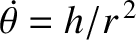, is given by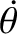(5.19)

For the case of attractive power-law central forces of the form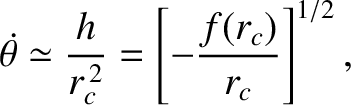, where, the apsidal angle becomes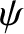(5.20)

It should be clear that if an orbit is going to close on itself then the apsidal angle needs to be a rational fraction of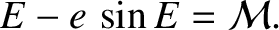. There are, in fact, only two small-integer values of the power-law index,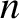, for which this is the case. As we have already seen, for an inverse-square force law (i.e.,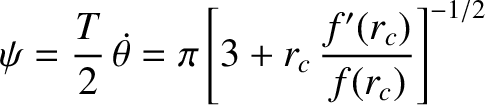), the apsidal angle is. Moreover, for a linear force law (i.e.,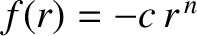), the apsidal angle is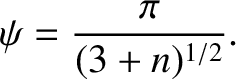. However, for quadratic (i.e.,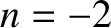) or cubic (i.e.,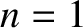) force laws, the apsidal angle is an irrational fraction of, which means that non-circular orbits in such force fields never close on themselves.Next: Perihelion precession of planets Up: Orbits in central force Previous: Motion in general central
Richard Fitzpatrick 2016-03-31